R Tutorial – We shall learn about R Operators – Arithmetic, Relational, Logical, Assignment and some of the Miscellaneous Operators that R programming language provides.

## R Operators

There are four main categories of Operators in R programming language. They are shown in the following picture :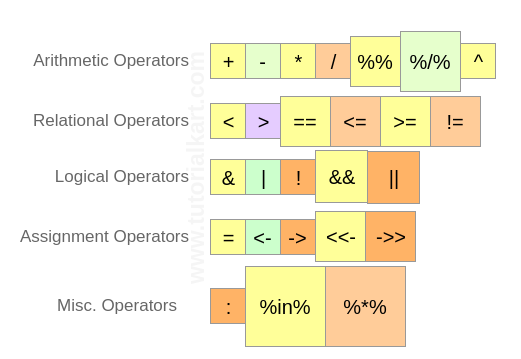We shall learn about these operators in detail with Example R programs.

## R Arithmetic Operators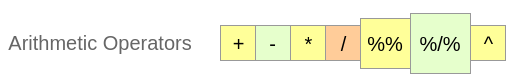Arithmetic Operators are used to accomplish arithmetic operations. They can be operated on the basic data types Numericals, Integers, Complex Numbers. Vectors with these basic data types can also participate in arithmetic operations, during which the operation is performed on one to one element basis.

 Operator Description Usage + Addition of two operands a + b – Subtraction of second operand from first a – b * Multiplication of two operands a * b / Division of first operand with second a / b %% Remainder from division of first operand with second a %% b %/% Quotient from division of first operand with second a %/% b ^ First operand raised to the power of second operand a^b

An example for each of the arithmetic operator on Numerical values is provided below :

An example for each of the arithmetic operator on Vectors is provided below :

## R Relational OperatorsRelational Operators are those that find out relation between the two operands provided to them. Following are the six relational operations R programming language supports.The output is boolean (TRUE or FALSE) for all of the Relational Operators in R programming language.

 Operator Description Usage < Is first operand less than second operand a < b > Is first operand greater than second operand a > b == Is first operand equal to second operand a == b <= Is first operand less than or equal to second operand a <= b >= Is first operand greater than or equal to second operand a > = b != Is first operand not equal to second operand a!=b

An example for each of the relational operator on Numberical values is provided below :

An example for each of the relational operator on Vectors is provided below :

## R Logical Operators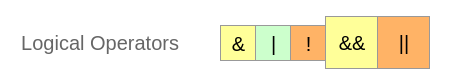Logical Operators in R programming language work only for the basic data types logical, numeric and complex and vectors of these basic data types.

 Operator Description Usage & Element wise logical AND operation. a & b | Element wise logical OR operation. a | b ! Element wise logical NOT operation. !a && Operand wise logical AND operation. a && b || Operand wise logical OR operation. a || b

An example for each of the logical operators on Numerical values is provided below :

An example for each of the logical operators on Vectors is provided below :

## R Assignment Operators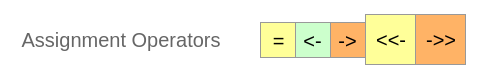Assignment Operators are those that help in assigning a value to the variable.

 Operator Description Usage = Assigns right side value to left side operand a = 3 <- Assigns right side value to left side operand a <- 5 -> Assigns left side value to right side operand 4 -> a <<- Assigns right side value to left side operand a <<- 3.4 ->> Assigns left side value to right side operand c(1,2) ->> a

An example for each of the assignment operators is provided below :

## R Miscellaneous Operators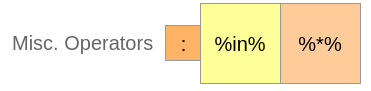These operators does not fall into any of the categories mentioned above, but are significantly important during R programming for manipulating data.

 Operator Description Usage : Creates series of numbers from left operand to right operand a:b %in% Identifies if an element(a) belongs to a vector(b) a %in% b %*% Performs multiplication of a vector with its transpose A %*% t(A)

An example for each of the Miscellaneous operators is provided below :

### Conclusion :

In this R Tutorial, we have learnt about R Operators – R Arithmetic Operators, R Relational Operators, R Logical Operators, R Assignment Operators, R Miscellaneous Operators with example R commands and R script files.